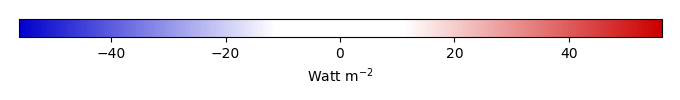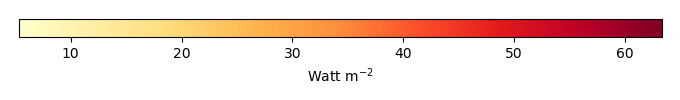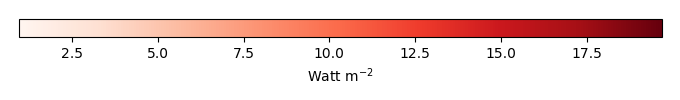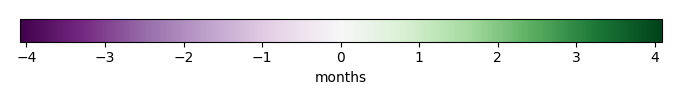# Mean State

Period Mean (original grids) [Watt m-2]
Model Period Mean (intersection) [Watt m-2]
Model Period Mean (complement) [Watt m-2]
Benchmark Period Mean (intersection) [Watt m-2]
Benchmark Period Mean (complement) [Watt m-2]
Bias [Watt m-2]
RMSE [Watt m-2]
Phase Shift [months]
Bias Score 
RMSE Score 
Seasonal Cycle Score 
Spatial Distribution Score 
Interannual Variability Score 
Overall Score 
Benchmark [-] 38.3
CLM4 [-] 34.6 34.4 0.00 39.6 15.5 -4.65 11.3 1.66 0.32 0.37 0.74 0.96 0.52 0.55
CLM4.5 [-] 34.2 33.9 0.00 39.6 15.5 -5.00 11.6 1.73 0.31 0.36 0.72 0.96 0.52 0.54
CLM5 [-] 33.9 33.6 0.00 39.6 15.5 -5.32 11.4 1.57 0.31 0.37 0.75 0.98 0.53 0.55
Period Mean (original grids) [Watt m-2]
Model Period Mean (intersection) [Watt m-2]
Model Period Mean (complement) [Watt m-2]
Benchmark Period Mean (intersection) [Watt m-2]
Benchmark Period Mean (complement) [Watt m-2]
Bias [Watt m-2]
RMSE [Watt m-2]
Phase Shift [months]
Bias Score 
RMSE Score 
Seasonal Cycle Score 
Spatial Distribution Score 
Interannual Variability Score 
Overall Score 
Benchmark [-] 52.3
CLM4 [-] 47.2 47.6 0.00 52.8 14.1 -5.33 13.1 1.28 0.44 0.37 0.83 0.99 0.56 0.59
CLM4.5 [-] 46.2 46.7 0.00 52.8 14.1 -6.19 13.5 1.19 0.41 0.37 0.85 0.99 0.56 0.59
CLM5 [-] 45.3 45.8 0.00 52.8 14.1 -7.07 13.8 1.27 0.40 0.38 0.85 0.99 0.53 0.59
Period Mean (original grids) [Watt m-2]
Model Period Mean (intersection) [Watt m-2]
Model Period Mean (complement) [Watt m-2]
Benchmark Period Mean (intersection) [Watt m-2]
Benchmark Period Mean (complement) [Watt m-2]
Bias [Watt m-2]
RMSE [Watt m-2]
Phase Shift [months]
Bias Score 
RMSE Score 
Seasonal Cycle Score 
Spatial Distribution Score 
Interannual Variability Score 
Overall Score 
Benchmark [-] 39.3
CLM4 [-] 37.3 37.4 0.00 40.4 16.6 -2.94 12.0 1.96 0.42 0.37 0.69 0.92 0.59 0.56
CLM4.5 [-] 36.8 36.8 0.00 40.4 16.6 -3.44 12.4 1.95 0.40 0.37 0.69 0.93 0.59 0.56
CLM5 [-] 36.6 36.6 0.00 40.4 16.6 -3.70 12.2 1.81 0.40 0.38 0.72 0.91 0.58 0.56
Period Mean (original grids) [Watt m-2]
Model Period Mean (intersection) [Watt m-2]
Model Period Mean (complement) [Watt m-2]
Benchmark Period Mean (intersection) [Watt m-2]
Benchmark Period Mean (complement) [Watt m-2]
Bias [Watt m-2]
RMSE [Watt m-2]
Phase Shift [months]
Bias Score 
RMSE Score 
Seasonal Cycle Score 
Spatial Distribution Score 
Interannual Variability Score 
Overall Score 
Benchmark [-] 31.6
CLM4 [-] 40.8 40.7 0.00 31.5 32.6 9.34 24.1 0.258 0.70 0.51 0.98 0.96 0.80 0.74
CLM4.5 [-] 35.8 35.7 0.00 31.5 32.6 5.05 19.2 0.264 0.79 0.57 0.98 0.97 0.75 0.77
CLM5 [-] 34.3 34.2 0.00 31.5 32.6 3.60 18.0 0.327 0.80 0.59 0.98 0.97 0.76 0.78
Period Mean (original grids) [Watt m-2]
Model Period Mean (intersection) [Watt m-2]
Model Period Mean (complement) [Watt m-2]
Benchmark Period Mean (intersection) [Watt m-2]
Benchmark Period Mean (complement) [Watt m-2]
Bias [Watt m-2]
RMSE [Watt m-2]
Phase Shift [months]
Bias Score 
RMSE Score 
Seasonal Cycle Score 
Spatial Distribution Score 
Interannual Variability Score 
Overall Score 
Benchmark [-] 23.5
CLM4 [-] 27.7 27.7 0.00 25.9 15.5 2.39 11.1 1.47 0.36 0.35 0.79 0.25 0.58 0.45
CLM4.5 [-] 27.5 27.5 0.00 25.9 15.5 2.24 11.1 1.47 0.35 0.36 0.79 0.28 0.57 0.45
CLM5 [-] 27.8 27.7 0.00 25.9 15.5 2.49 11.1 1.43 0.36 0.36 0.79 0.23 0.55 0.44
Period Mean (original grids) [Watt m-2]
Model Period Mean (intersection) [Watt m-2]
Model Period Mean (complement) [Watt m-2]
Benchmark Period Mean (intersection) [Watt m-2]
Benchmark Period Mean (complement) [Watt m-2]
Bias [Watt m-2]
RMSE [Watt m-2]
Phase Shift [months]
Bias Score 
RMSE Score 
Seasonal Cycle Score 
Spatial Distribution Score 
Interannual Variability Score 
Overall Score 
Benchmark [-] 21.4
CLM4 [-] 23.8 23.7 0.00 21.5 17.6 2.22 11.2 0.647 0.76 0.50 0.92 0.86 0.73 0.71
CLM4.5 [-] 26.0 25.9 0.00 21.5 17.6 4.39 13.4 0.534 0.69 0.46 0.94 0.73 0.72 0.67
CLM5 [-] 25.4 25.3 0.00 21.5 17.6 3.82 12.6 0.569 0.73 0.47 0.93 0.82 0.74 0.69
Period Mean (original grids) [Watt m-2]
Model Period Mean (intersection) [Watt m-2]
Model Period Mean (complement) [Watt m-2]
Benchmark Period Mean (intersection) [Watt m-2]
Benchmark Period Mean (complement) [Watt m-2]
Bias [Watt m-2]
RMSE [Watt m-2]
Phase Shift [months]
Bias Score 
RMSE Score 
Seasonal Cycle Score 
Spatial Distribution Score 
Interannual Variability Score 
Overall Score 
Benchmark [-] 30.0
CLM4 [-] 36.2 36.1 0.00 30.5 14.9 5.01 11.9 0.933 0.64 0.48 0.88 0.91 0.66 0.67
CLM4.5 [-] 37.0 37.0 0.00 30.5 14.9 5.79 12.3 0.946 0.61 0.47 0.88 0.91 0.66 0.67
CLM5 [-] 35.3 35.3 0.00 30.5 14.9 4.16 11.4 0.960 0.67 0.47 0.87 0.97 0.67 0.69
Period Mean (original grids) [Watt m-2]
Model Period Mean (intersection) [Watt m-2]
Model Period Mean (complement) [Watt m-2]
Benchmark Period Mean (intersection) [Watt m-2]
Benchmark Period Mean (complement) [Watt m-2]
Bias [Watt m-2]
RMSE [Watt m-2]
Phase Shift [months]
Bias Score 
RMSE Score 
Seasonal Cycle Score 
Spatial Distribution Score 
Interannual Variability Score 
Overall Score 
Benchmark [-] 29.5
CLM4 [-] 34.3 34.2 0.00 30.6 14.2 3.13 12.3 0.998 0.58 0.38 0.89 0.96 0.57 0.63
CLM4.5 [-] 33.9 33.8 0.00 30.6 14.2 2.71 12.2 0.978 0.57 0.38 0.89 0.97 0.54 0.62
CLM5 [-] 33.5 33.4 0.00 30.6 14.2 2.29 11.6 0.992 0.59 0.40 0.88 0.98 0.51 0.63
Period Mean (original grids) [Watt m-2]
Model Period Mean (intersection) [Watt m-2]
Model Period Mean (complement) [Watt m-2]
Benchmark Period Mean (intersection) [Watt m-2]
Benchmark Period Mean (complement) [Watt m-2]
Bias [Watt m-2]
RMSE [Watt m-2]
Phase Shift [months]
Bias Score 
RMSE Score 
Seasonal Cycle Score 
Spatial Distribution Score 
Interannual Variability Score 
Overall Score 
Benchmark [-] 33.0
CLM4 [-] 37.8 37.6 0.00 32.8 37.1 4.93 21.2 0.212 0.79 0.55 0.99 0.82 0.79 0.75
CLM4.5 [-] 35.1 34.9 0.00 32.8 37.1 2.57 19.1 0.244 0.81 0.58 0.98 0.90 0.74 0.77
CLM5 [-] 32.3 32.2 0.00 32.8 37.1 -0.0780 17.2 0.288 0.82 0.61 0.98 0.85 0.73 0.77
Period Mean (original grids) [Watt m-2]
Model Period Mean (intersection) [Watt m-2]
Model Period Mean (complement) [Watt m-2]
Benchmark Period Mean (intersection) [Watt m-2]
Benchmark Period Mean (complement) [Watt m-2]
Bias [Watt m-2]
RMSE [Watt m-2]
Phase Shift [months]
Bias Score 
RMSE Score 
Seasonal Cycle Score 
Spatial Distribution Score 
Interannual Variability Score 
Overall Score 
Benchmark [-] 35.1
CLM4 [-] 41.0 41.0 0.00 36.7 14.1 3.17 10.4 0.491 0.60 0.54 0.96 0.92 0.61 0.69
CLM4.5 [-] 41.0 41.0 0.00 36.7 14.1 3.20 10.4 0.466 0.61 0.54 0.96 0.94 0.60 0.70
CLM5 [-] 39.7 39.8 0.00 36.7 14.1 2.08 10.6 0.510 0.58 0.53 0.96 0.89 0.65 0.69
Period Mean (original grids) [Watt m-2]
Model Period Mean (intersection) [Watt m-2]
Model Period Mean (complement) [Watt m-2]
Benchmark Period Mean (intersection) [Watt m-2]
Benchmark Period Mean (complement) [Watt m-2]
Bias [Watt m-2]
RMSE [Watt m-2]
Phase Shift [months]
Bias Score 
RMSE Score 
Seasonal Cycle Score 
Spatial Distribution Score 
Interannual Variability Score 
Overall Score 
Benchmark [-] 19.8
CLM4 [-] 23.5 23.5 0.00 20.7 10.0 2.65 11.6 0.885 0.68 0.51 0.91 0.97 0.67 0.71
CLM4.5 [-] 22.4 22.4 0.00 20.7 10.0 1.68 10.4 1.14 0.71 0.52 0.86 0.90 0.70 0.70
CLM5 [-] 22.5 22.5 0.00 20.7 10.0 1.77 10.1 1.13 0.71 0.53 0.87 0.87 0.68 0.70
Period Mean (original grids) [Watt m-2]
Model Period Mean (intersection) [Watt m-2]
Model Period Mean (complement) [Watt m-2]
Benchmark Period Mean (intersection) [Watt m-2]
Benchmark Period Mean (complement) [Watt m-2]
Bias [Watt m-2]
RMSE [Watt m-2]
Phase Shift [months]
Bias Score 
RMSE Score 
Seasonal Cycle Score 
Spatial Distribution Score 
Interannual Variability Score 
Overall Score 
Benchmark [-] 23.6
CLM4 [-] 46.3 46.3 0.00 43.1 14.1 2.21 15.1 0.855 0.62 0.49 0.89 0.87 0.61 0.66
CLM4.5 [-] 45.6 45.6 0.00 43.1 14.1 1.62 14.6 0.863 0.61 0.49 0.89 0.85 0.60 0.65
CLM5 [-] 45.0 45.0 0.00 43.1 14.1 1.14 14.4 0.858 0.62 0.49 0.89 0.88 0.60 0.66
Period Mean (original grids) [Watt m-2]
Model Period Mean (intersection) [Watt m-2]
Model Period Mean (complement) [Watt m-2]
Benchmark Period Mean (intersection) [Watt m-2]
Benchmark Period Mean (complement) [Watt m-2]
Bias [Watt m-2]
RMSE [Watt m-2]
Phase Shift [months]
Bias Score 
RMSE Score 
Seasonal Cycle Score 
Spatial Distribution Score 
Interannual Variability Score 
Overall Score 
Benchmark [-] 64.3
CLM4 [-] 80.2 80.0 0.00 66.2 16.5 12.1 19.4 0.472 0.54 0.58 0.96 0.99 0.51 0.69
CLM4.5 [-] 79.8 79.6 0.00 66.2 16.5 11.6 19.1 0.477 0.55 0.58 0.96 0.99 0.52 0.69
CLM5 [-] 78.4 78.2 0.00 66.2 16.5 10.2 18.6 0.552 0.56 0.57 0.95 0.99 0.54 0.70
Period Mean (original grids) [Watt m-2]
Model Period Mean (intersection) [Watt m-2]
Model Period Mean (complement) [Watt m-2]
Benchmark Period Mean (intersection) [Watt m-2]
Benchmark Period Mean (complement) [Watt m-2]
Bias [Watt m-2]
RMSE [Watt m-2]
Phase Shift [months]
Bias Score 
RMSE Score 
Seasonal Cycle Score 
Spatial Distribution Score 
Interannual Variability Score 
Overall Score 
Benchmark [-] 34.3
CLM4 [-] 40.6 40.6 0.00 34.2 35.0 7.15 21.0 0.196 0.75 0.57 0.98 0.96 0.81 0.77
CLM4.5 [-] 37.6 37.5 0.00 34.2 35.0 4.54 18.1 0.214 0.79 0.61 0.98 0.96 0.77 0.79
CLM5 [-] 37.5 37.5 0.00 34.2 35.0 4.47 18.3 0.207 0.79 0.61 0.98 0.98 0.77 0.79
Period Mean (original grids) [Watt m-2]
Model Period Mean (intersection) [Watt m-2]
Model Period Mean (complement) [Watt m-2]
Benchmark Period Mean (intersection) [Watt m-2]
Benchmark Period Mean (complement) [Watt m-2]
Bias [Watt m-2]
RMSE [Watt m-2]
Phase Shift [months]
Bias Score 
RMSE Score 
Seasonal Cycle Score 
Spatial Distribution Score 
Interannual Variability Score 
Overall Score 
Benchmark [-] 24.8
CLM4 [-] 34.1 34.1 0.00 27.0 14.3 6.50 11.1 0.817 0.50 0.43 0.92 0.95 0.61 0.64
CLM4.5 [-] 34.3 34.3 0.00 27.0 14.3 6.96 11.3 0.852 0.52 0.42 0.91 0.91 0.61 0.63
CLM5 [-] 33.8 33.8 0.00 27.0 14.3 6.58 11.1 0.872 0.52 0.44 0.91 0.85 0.59 0.62
Period Mean (original grids) [Watt m-2]
Model Period Mean (intersection) [Watt m-2]
Model Period Mean (complement) [Watt m-2]
Benchmark Period Mean (intersection) [Watt m-2]
Benchmark Period Mean (complement) [Watt m-2]
Bias [Watt m-2]
RMSE [Watt m-2]
Phase Shift [months]
Bias Score 
RMSE Score 
Seasonal Cycle Score 
Spatial Distribution Score 
Interannual Variability Score 
Overall Score 
Benchmark [-] 20.7
CLM4 [-] 25.7 25.5 0.00 21.1 12.1 4.25 8.93 0.877 0.67 0.48 0.89 0.98 0.61 0.68
CLM4.5 [-] 26.5 26.3 0.00 21.1 12.1 5.05 9.20 0.834 0.61 0.47 0.89 0.99 0.68 0.69
CLM5 [-] 27.4 27.3 0.00 21.1 12.1 6.00 9.62 0.994 0.56 0.48 0.87 0.99 0.65 0.67
Period Mean (original grids) [Watt m-2]
Model Period Mean (intersection) [Watt m-2]
Model Period Mean (complement) [Watt m-2]
Benchmark Period Mean (intersection) [Watt m-2]
Benchmark Period Mean (complement) [Watt m-2]
Bias [Watt m-2]
RMSE [Watt m-2]
Phase Shift [months]
Bias Score 
RMSE Score 
Seasonal Cycle Score 
Spatial Distribution Score 
Interannual Variability Score 
Overall Score 
Benchmark [-] 20.3
CLM4 [-] 23.7 23.8 0.00 20.6 10.6 3.09 10.9 0.587 0.67 0.52 0.92 0.79 0.66 0.68
CLM4.5 [-] 23.1 23.2 0.00 20.6 10.6 2.50 10.3 0.625 0.69 0.52 0.92 0.88 0.64 0.70
CLM5 [-] 23.7 23.7 0.00 20.6 10.6 3.05 10.4 0.622 0.68 0.53 0.92 0.90 0.67 0.70
Period Mean (original grids) [Watt m-2]
Model Period Mean (intersection) [Watt m-2]
Model Period Mean (complement) [Watt m-2]
Benchmark Period Mean (intersection) [Watt m-2]
Benchmark Period Mean (complement) [Watt m-2]
Bias [Watt m-2]
RMSE [Watt m-2]
Phase Shift [months]
Bias Score 
RMSE Score 
Seasonal Cycle Score 
Spatial Distribution Score 
Interannual Variability Score 
Overall Score 
Benchmark [-] 30.8
CLM4 [-] 35.9 35.8 0.00 31.1 10.8 4.33 10.6 0.729 0.65 0.50 0.90 0.96 0.68 0.70
CLM4.5 [-] 37.0 37.0 0.00 31.1 10.8 5.45 10.8 0.739 0.60 0.51 0.90 0.94 0.71 0.70
CLM5 [-] 34.2 34.2 0.00 31.1 10.8 2.76 9.44 0.757 0.70 0.51 0.90 0.99 0.73 0.73
Period Mean (original grids) [Watt m-2]
Model Period Mean (intersection) [Watt m-2]
Model Period Mean (complement) [Watt m-2]
Benchmark Period Mean (intersection) [Watt m-2]
Benchmark Period Mean (complement) [Watt m-2]
Bias [Watt m-2]
RMSE [Watt m-2]
Phase Shift [months]
Bias Score 
RMSE Score 
Seasonal Cycle Score 
Spatial Distribution Score 
Interannual Variability Score 
Overall Score 
Benchmark [-] 26.8
CLM4 [-] 27.2 27.2 0.00 27.1 15.2 0.203 8.39 2.23 0.73 0.36 0.65 0.82 0.47 0.56
CLM4.5 [-] 26.9 26.9 0.00 27.1 15.2 -0.0413 8.43 2.24 0.73 0.36 0.65 0.84 0.47 0.57
CLM5 [-] 27.1 27.1 0.00 27.1 15.2 0.130 8.33 2.25 0.74 0.37 0.64 0.81 0.45 0.56
Period Mean (original grids) [Watt m-2]
Model Period Mean (intersection) [Watt m-2]
Model Period Mean (complement) [Watt m-2]
Benchmark Period Mean (intersection) [Watt m-2]
Benchmark Period Mean (complement) [Watt m-2]
Bias [Watt m-2]
RMSE [Watt m-2]
Phase Shift [months]
Bias Score 
RMSE Score 
Seasonal Cycle Score 
Spatial Distribution Score 
Interannual Variability Score 
Overall Score 
Benchmark [-] 29.5
CLM4 [-] 30.9 30.9 0.00 29.9 14.5 1.03 9.09 1.38 0.74 0.43 0.81 0.88 0.56 0.64
CLM4.5 [-] 31.0 31.1 0.00 29.9 14.5 1.15 9.05 1.45 0.76 0.43 0.80 0.87 0.56 0.64
CLM5 [-] 31.6 31.6 0.00 29.9 14.5 1.66 8.89 1.03 0.74 0.44 0.86 0.81 0.53 0.64
Period Mean (original grids) [Watt m-2]
Model Period Mean (intersection) [Watt m-2]
Model Period Mean (complement) [Watt m-2]
Benchmark Period Mean (intersection) [Watt m-2]
Benchmark Period Mean (complement) [Watt m-2]
Bias [Watt m-2]
RMSE [Watt m-2]
Phase Shift [months]
Bias Score 
RMSE Score 
Seasonal Cycle Score 
Spatial Distribution Score 
Interannual Variability Score 
Overall Score 
Benchmark [-] 28.0
CLM4 [-] 35.1 35.2 0.00 29.7 12.6 4.88 12.6 0.682 0.56 0.48 0.92 0.96 0.57 0.66
CLM4.5 [-] 34.5 34.5 0.00 29.7 12.6 4.29 12.3 0.686 0.56 0.48 0.92 0.95 0.58 0.66
CLM5 [-] 33.1 33.1 0.00 29.7 12.6 2.96 12.0 0.745 0.57 0.48 0.92 0.93 0.58 0.66

# Temporally integrated period mean

BENCHMARK MEANMODEL MEANBIASBIAS SCORERMSERMSE SCOREBENCHMARK INTERANNUAL VARIABILITYMODEL INTERANNUAL VARIABILITYINTERANNUAL VARIABILITY SCOREBENCHMARK MAX MONTHMODEL MAX MONTHDIFFERENCE IN MAX MONTHSEASONAL CYCLE SCORESPATIAL TAYLOR DIAGRAMMODEL COLORS# Spatially integrated regional mean

MODEL COLORSREGIONAL MEANANNUAL CYCLEMONTHLY ANOMALYANNUAL CYCLE# All Models

BenchmarkCLM4CLM4.5CLM5# Data Information

creation_date: Mon Jun 30 23:28:16 PDT 2014

source_file: This product is generated from monthly 1 degree GEWEX SRB Radiation observations